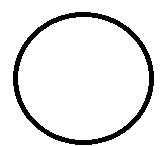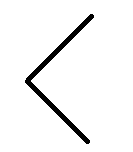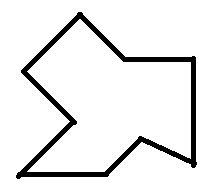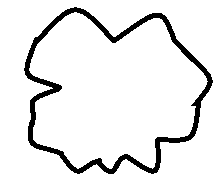QuestionAnswers

# Draw rough diagram to illustrate the following, Closed curve.Verified
128.7k+ views
Hint: Quadrilaterals are one type of polygon that has four sides and four vertices and four angles along with 2 diagonals. There are various types of quadrilaterals. Trapezium, kite, square, etc.

Introduction of curves-
A curve is a geometrical figure obtained when a number of points are joined without lifting the pencil from the paper and without retracing any portion. It is basically a need which not to be straight.
The various types of curves are:
Open curve- An open curve is a curve in which there is no path from any of its point to the same point.
Closed curve- This is a curve that forms a path any of its point to the same point.
A curve can be
-a closed curve-an open curve-a closed curve which is not simple.Therefore,